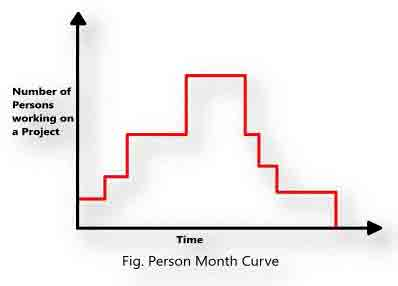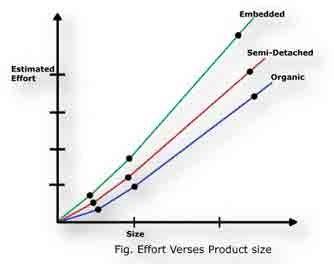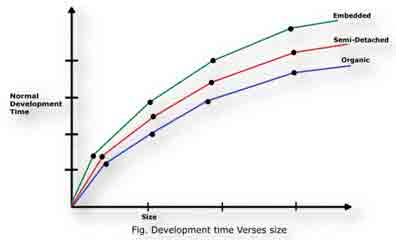# Software Engineering (2160701)

BE | Semester-6   Summer-2018 | 04/28/2018

## Q2) (b)

#### Explain COCOMO model for project estimation

• COCOMO (Constructive Cost Estimation Model) was proposed by Boehm
• According to Boehm, software cost estimation should be done through three stages:
• Basic COCOMO,
• Intermediate COCOMO, and
• Complete COCOMO

#### Basic COCOMO Model

• The basic COCOMO model gives an approximate estimate of the project parameters
• The basic COCOMO estimation model is given by the following expressions
• Effort=a1+(KLOC)a2 PM  |  Tdev=b1*(Effort)b2 Months
• KLOC is the estimated size of the software product expressed in Kilo Lines of Code,
• a1, a2, b1, b2 are constants for each category of software products,
• Tdev is the estimated time to develop the software, expressed in months,
• Effort is the total effort required to develop the software product, expressed in person months (PMs).
Project a1 a2 b1 b2
Organic 2.5 1.05 2.5 0.38
Semidetached 3.0 1.12 2.5 0.35
Embedded 3.6 1.20 2.5 0.32

• The effort estimation is expressed in units of person-months (PM)
• It is the area under the person-month plot (as shown in fig.)
• An effort of 100 PM
• does not imply that 100 persons should work for 1 month
• does not imply that 1 person should be employed for 100 months
• it denotes the area under the person-month curve (fig.)(Figure: Person Month Curve)
• Every line of source text should be calculated as one LOC irrespective of the actual number of instructions on that line
• If a single instruction spans several lines (say n lines), it is considered to be nLOC
• The values of a1, a2, b1, b2 for different categories of products (i.e. organic, semidetached, and embedded) as given by Boehm
• He derived the expressions by examining historical data collected from a large number of actual projects
• Insight into the basic COCOMO model can be obtained by plotting the estimated characteristics for different software sizes
• Fig. shows a plot of estimated effort versus product size
• From fig. we can observe that the effort is somewhat superlinear in the size of the software product
• The effort required to develop a product increases very rapidly with project size(Figure: Efforts vs Product Size)
• The development time versus the product size in KLOC is plotted in fig.
• From fig., it can be observed that the development time is a sublinear function of the size of the product
• i.e. when the size of the product increases by two times, the time to develop the product does not double but rises moderately
• From fig., it can be observed that the development time is roughly the same for all the three categories of products(Figure: Development Time vs Size)
• Effort and the duration estimations obtained using the COCOMO model are called as nominal effort estimate and nominal duration estimate
• The term nominal implies that
• if anyone tries to complete the project in a time shorter than the estimated duration, then the cost will increase drastically
• But, if anyone completes the project over a longer period of time than the estimated, then there is almost no decrease in the estimated cost value